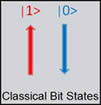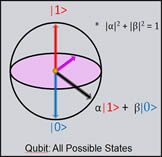# Introduction

### Quantum Information/ Computation

Quantum computation allows exponential speedup over classical computation for certain algorithms. In order to achieve this feat, a quantum computer exploits the fact that quantum bits (qubits) have a larger set of possible states than classical bits.

#### Classical bits vs Quantum Bits (Qubits)

 While classical bits can only be in states 0 or 1 , a qubit can be in a general superposition state ( a|0> + b|1> ). Two qubits can be placed in an entangled state,e.g. : ( 00 + 11) : either both qubits are in state 0 or both 1; outcome is only known when a measurement is made.#### Quantum Information Processing Requirements

The following requirements must be met for quantum computation [D. DiVicenzo; arXiv:quant-ph/0002077 ]:

1. Good qubits
2. Initialization of qubits in well-defined states
3. State- rotation of each bit
4. Entanglement of two different bits
5. Read-out of final state of qubits
6. Long decoherence times compared to time for gate operations